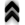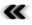## Appendix AResolvent for Integral Equations

While the computation of integrals in itself is a worthy application of Monte Carlo theory, especially in the case of high dimensionality of the integration domain, it can be employed to obtain solutions, or at least estimates, to integral equations.

Recalling that Fredholm integral equations of the second kind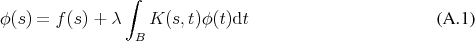have a solution of the form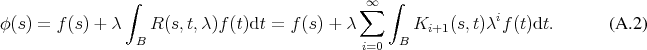as outlined in Section 4.9. This resolvent series includes repeated integrals which are accessible to Monte Carlo integration methods.

Beginning with examining the integrals in the resolvent series and rewriting them as expectation values gives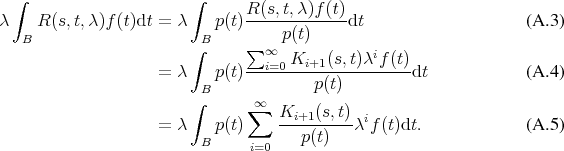Examining the terms in order of increasing summation index shows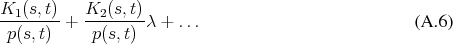Recalling Equation 4.184 gives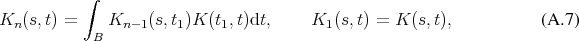where further integrals appear, when expanding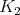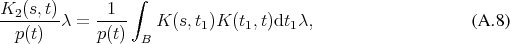which can again be evaluated in a similar fashion as an expectation value (Deﬁnition 101) with a random variable (Deﬁnition 98).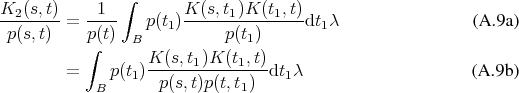The recursion relation A.7 allows to reuse the obtained result in the subsequent terms and further recursion reveals that the term for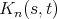evaluated using Monte Carlo methods (see Section 6.6.2) takes the shape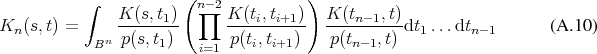Thus every term in the series requires an additional random number to be generated in order to calculate an expectation value. The notion of the procedure is depicted in Figure A.1.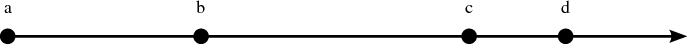Figure A.1: The general Monte Carlo scheme due to Equation A.10.